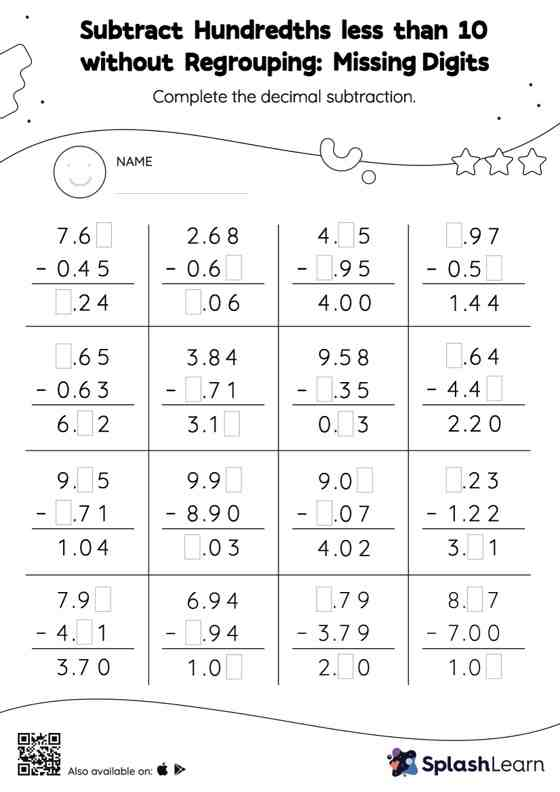# Subtract Hundredths less than 10 without Regrouping: Missing Digits Worksheet

Home > Subtract Hundredths less than 10 without Regrouping: Missing DigitsThis subtract hundredths less than 10 without regrouping worksheet has a set of problems curated to ensure that your child becomes more proficient in subtraction. While subtracting decimals, students use the relationship between addition and subtraction to find the missing number. They do not need to regroup numbers in subtract hundredths less than 10 without regrouping worksheet.